HomeCompositio Mathematica

# Sub-Weyl subconvexity for Dirichlet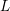$L$ -functions to prime power moduli

Published online by Cambridge University Press:  03 November 2015

Corresponding

## Abstract

We prove a subconvexity bound for the central value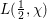$L(\frac{1}{2},{\it\chi})$ of a Dirichlet$L$ -function of a character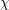${\it\chi}$ to a prime power modulus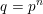$q=p^{n}$ of the form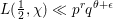$L(\frac{1}{2},{\it\chi})\ll p^{r}q^{{\it\theta}+{\it\epsilon}}$ with a fixed$r$ and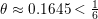${\it\theta}\approx 0.1645<\frac{1}{6}$ , breaking the long-standing Weyl exponent barrier. In fact, we develop a general new theory of estimation of short exponential sums involving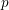$p$ -adically analytic phases, which can be naturally seen as a$p$ -adic analogue of the method of exponent pairs. This new method is presented in a ready-to-use form and applies to a wide class of well-behaved phases including many that arise from a stationary phase analysis of hyper-Kloosterman and other complete exponential sums.

## MSC classification

Type
Research Article
Information
Compositio Mathematica , April 2016 , pp. 825 - 875

## Access options

Get access to the full version of this content by using one of the access options below.

## References

Barban, M. B., Linnik, Yu. V. and Tshudakov, N. G., On prime numbers in an arithmetic progression with a prime-power difference, Acta Arith. 9 (1964), 375390; MR 0171766 (30 #1993).CrossRefGoogle Scholar
Berndt, B. C., Evans, R. J. and Williams, K. S., Gauss and Jacobi sums, Canadian Mathematical Society Series of Monographs and Advanced Texts (Wiley, New York, 1998); MR 1625181 (99d:11092).Google Scholar
Blomer, V. and Milićević, D., p-adic analytic twists and strong subconvexity, Ann. Sci. Éc. Norm. Supér. (4) 48 (2015), 561605; MR 3377053.CrossRefGoogle Scholar
Blomer, V. and Milićević, D., The second moment of twisted modular L-functions, Geom. Funct. Anal. 25 (2015), 453516; doi: 10.1007/s00039-015-0318-7; MR 3334233.CrossRefGoogle Scholar
Bourgain, J., Decoupling, exponential sums and the Riemann zeta function, Preprint (2014),arXiv:1408.5794.Google Scholar
Burgess, D. A., On character sums and L-series. II, Proc. Lond. Math. Soc. (3) 13 (1963), 524536; MR 0148626 (26 #6133).CrossRefGoogle Scholar
Conrey, J. B. and Iwaniec, H., The cubic moment of central values of automorphic L-functions, Ann. of Math. (2) 151 (2000), 11751216; MR 1779567 (2001g:11070).CrossRefGoogle Scholar
Fujii, A., Gallagher, P. X. and Montgomery, H. L., Some hybrid bounds for character sums and Dirichlet L-series, in Topics in number theory (Proc. Colloq., Debrecen, 1974), Colloq. Math. Soc. János Bolyai, vol. 13 (North-Holland, Amsterdam, 1976), 4157; MR 0434987 (55 #7949).Google Scholar
Gallagher, P. X., Primes in progressions to prime-power modulus, Invent. Math. 16 (1972), 191201; MR 0304327 (46 #3462).CrossRefGoogle Scholar
Graham, S. W. and Kolesnik, G., Van der Corput’s method of exponential sums, London Mathematical Society Lecture Note Series, vol. 126 (Cambridge University Press, Cambridge, 1991); MR 1145488 (92k:11082).Google Scholar
Heath-Brown, D. R., Hybrid bounds for Dirichlet L-functions, Invent. Math. 47 (1978), 149170; MR 0485727 (58 #5549).CrossRefGoogle Scholar
Hiary, G. A., Computing Dirichlet character sums to a power-full modulus, J. Number Theory 140 (2014), 122146; MR 3181649.CrossRefGoogle Scholar
Holowinsky, R., Munshi, R. and Qi, Z., Character sums of composite moduli and hybrid subconvexity, Contemp. Math., to appear. Preprint (2014), arXiv:1409.3797.Google Scholar
Huxley, M. N., Exponential sums and the Riemann zeta function. V, Proc. Lond. Math. Soc. (3) 90 (2005), 141; MR 2107036 (2005h:11180).CrossRefGoogle Scholar
Iwaniec, H., On zeros of Dirichlet’s L series, Invent. Math. 23 (1974), 97104; MR 0344207 (49 #8947).CrossRefGoogle Scholar
Iwaniec, H. and Kowalski, E., Analytic number theory, American Mathematical Society Colloquium Publications, vol. 53 (American Mathematical Society, Providence, RI, 2004); MR 2061214 (2005h:11005).Google Scholar
Iwaniec, H. and Sarnak, P., Perspectives on the analytic theory of L-functions, Geom. Funct. Anal. (2000), Special Volume, Part II, 705–741, GAFA 2000 (Tel Aviv, 1999); MR 1826269 (2002b:11117).Google Scholar
Katok, S., p-adic analysis compared with real, Student Mathematical Library, vol. 37 (American Mathematical Society, Providence, RI, 2007); MR 2298943 (2008j:12010).Google Scholar
Michel, P., Analytic number theory and families of automorphic L-functions, in Automorphic forms and applications, IAS/Park City Mathematics Series, vol. 12 (American Mathematical Society, Providence, RI, 2007), 181295; MR 2331346 (2008m:11104).Google Scholar
Michel, P. and Venkatesh, A., The subconvexity problem for GL2, Publ. Math. Inst. Hautes Études Sci. 111 (2010), 171271; MR 2653249.CrossRefGoogle Scholar
Nelson, P. D., Pitale, A. and Saha, A., Bounds for Rankin–Selberg integrals and quantum unique ergodicity for powerful levels, J. Amer. Math. Soc. 27 (2014), 147191; MR 3110797.CrossRefGoogle Scholar
Phillips, E., The zeta-function of Riemann; further developments of van der Corput’s method, Q. J. Math. 4 (1933), 209225.CrossRefGoogle Scholar
Postnikov, A. G., On the sum of characters with respect to a modulus equal to a power of a prime number, Izv. Akad. Nauk SSSR. Ser. Mat. 19 (1955), 1116; MR 0068575 (16,905f).Google Scholar
Rankin, R. A., Van der Corput’s method and the theory of exponent pairs, Q. J. Math. 6 (1955), 147153; MR 0072170 (17,240a).CrossRefGoogle Scholar
Ricotta, G., Universality of convexity breaking exponents, in Problem Sessions: Subconvexity Bounds for$L$-functions (notes), 2006,http://www.aimath.org/WWN/subconvexity/subconvexity.pdf.Google Scholar
Robert, A. M., A course in p-adic analysis, Graduate Texts in Mathematics, vol. 198 (Springer, New York, 2000); MR 1760253 (2001g:11182).Google Scholar
Salié, H., Über die Kloostermanschen Summen S (u, v; q), Math. Z. 34 (1932), 91109; MR 1545243.CrossRefGoogle Scholar
Templier, N., Large values of modular forms, Camb. J. Math. 2 (2014), 91116; MR 3272013.CrossRefGoogle Scholar
Titchmarsh, E. C., The theory of the Riemann zeta-function, 2nd edition (The Clarendon Press, Oxford University Press, New York, 1986), Edited and with a preface by D. R. Heath-Brown; MR 882550 (88c:11049).Google Scholar
van der Corput, J. G., Verschärfung der Abschätzung beim Teilerproblem, Math. Ann. 87 (1922), 3965.CrossRefGoogle Scholar
Vishe, P., A fast algorithm to compute L (1∕2, f ×𝜒q), J. Number Theory 133 (2013), 15021524; MR 3007119.CrossRefGoogle Scholar
Walfisz, A., Zur Abschätzung von 𝜁(1∕2 + it), Nachr. Ges. Wiss. Göttingen Math.-Physik. Kl. 1924 (1924), 155158 (in German).Google Scholar

### Full text views

Full text views reflects PDF downloads, PDFs sent to Google Drive, Dropbox and Kindle and HTML full text views.

Total number of HTML views: 0
Total number of PDF views: 133 *
View data table for this chart

* Views captured on Cambridge Core between September 2016 - 17th January 2021. This data will be updated every 24 hours.

Hostname: page-component-77fc7d77f9-2nq4t Total loading time: 0.319 Render date: 2021-01-17T10:07:48.375Z Query parameters: { "hasAccess": "0", "openAccess": "0", "isLogged": "0", "lang": "en" } Feature Flags last update: Sun Jan 17 2021 10:02:28 GMT+0000 (Coordinated Universal Time) Feature Flags: { "metrics": true, "metricsAbstractViews": false, "peerReview": true, "crossMark": true, "comments": true, "relatedCommentaries": true, "subject": true, "clr": true, "languageSwitch": true, "figures": false, "newCiteModal": false, "shouldUseShareProductTool": true, "shouldUseHypothesis": true, "isUnsiloEnabled": true }

# Send article to Kindle

Note you can select to send to either the @free.kindle.com or @kindle.com variations. ‘@free.kindle.com’ emails are free but can only be sent to your device when it is connected to wi-fi. ‘@kindle.com’ emails can be delivered even when you are not connected to wi-fi, but note that service fees apply.

Find out more about the Kindle Personal Document Service.

Sub-Weyl subconvexity for Dirichlet$L$-functions to prime power moduli Available formats × # Send article to Dropbox To send this article to your Dropbox account, please select one or more formats and confirm that you agree to abide by our usage policies. If this is the first time you use this feature, you will be asked to authorise Cambridge Core to connect with your <service> account. Find out more about sending content to Dropbox. Sub-Weyl subconvexity for Dirichlet$L$ -functions to prime power moduli
Available formats
×

# Send article to Google Drive

To send this article to your Google Drive account, please select one or more formats and confirm that you agree to abide by our usage policies. If this is the first time you use this feature, you will be asked to authorise Cambridge Core to connect with your <service> account. Find out more about sending content to Google Drive.

Sub-Weyl subconvexity for Dirichlet$L\$ -functions to prime power moduli
Available formats
×
×